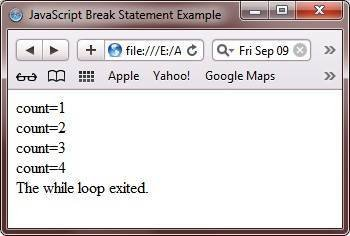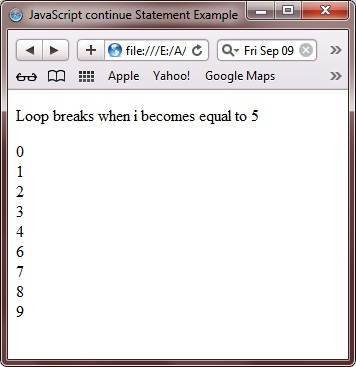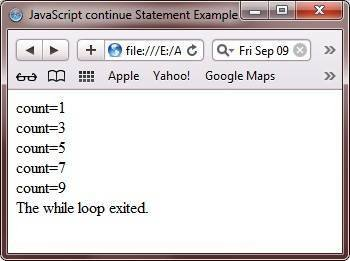# JavaScript Break and Continue

JavaScript break and continue statement/keyword is used to control the loop.

## JavaScript break Statement

JavaScript break statement is used to exit from the loop when the required condition is met. Here is an example uses break statement to exit the loop:

```<!DOCTYPE html>
<html>
<head>
<title>JavaScript Break Statement</title>
</head>
<body>

<p>Loop breaks when i becomes equal to 5</p>
<p id="break_statement_para1"></p>
<script>
var text = "";
var i;
for(i = 0; i < 10; i++)
{
if(i === 5)
{
break;
}
text += + i + "<br>";
}
document.getElementById("break_statement_para1").innerHTML = text;
</script>

</body>
</html>```

Here is the output produced by the above JavaScript break statement example program.Here is the live demo output of the above break keyword example in JavaScript.

Loop breaks when i becomes equal to 5

Here is another example also demonstrates the use of break statement/keyword in JavaScript.

```<!DOCTYPE HTML>
<HTML>
<HEAD>
<TITLE>JavaScript Break Statement Example</TITLE>
</HEAD>
<BODY>

<SCRIPT type="text/javascript">
var count=0;
while(count<10)
{
++count;
if((count%5==0))
break;
else
document.write("count="+count+"<BR/>");
}
document.write("The while loop exited.");
</SCRIPT>

</BODY>
</HTML>```

Here is the output produced by the above break example in JavaScript.## JavaScript continue Statement

JavaScript continue statement is used to skip the remaining part of code and goes to the condition-checker part, if the required condition is met. Here is an example uses continue statement to skip one iteration of the loop:

```<!DOCTYPE html>
<html>
<head>
<title>JavaScript continue Statement Example</title>
</head>
<body>

<p>Loop breaks when i becomes equal to 5</p>
<p id="continue_statement_para1"></p>
<script>
var text = "";
var i;
for(i = 0; i < 10; i++)
{
if(i === 5)
{
continue;
}
text += + i + "<br>";
}
document.getElementById("continue_statement_para1").innerHTML = text;
</script>

</body>
</html>```

It will skip to print the value 5, so it will display the following result:Here is the live demo output of the above continue statement example program in JavaScript.

Loop breaks when i becomes equal to 5

Here is another example also demonstrates continue statement in JavaScript.

```<!DOCTYPE HTML>
<HTML>
<HEAD>
<TITLE>JavaScript continue Statement Example</TITLE>
</HEAD>
<BODY>

<SCRIPT type="text/javascript">
var count=0;
while(count<10)
{
++count;
if(count%2==0)
continue;
else
document.write("count="+count+"<BR/>");
}
document.write("The while loop exited.");
</SCRIPT>

</BODY>
</HTML>```

Here is the output of the above continue statement program in JavaScript.Tools
Calculator

Quick Links
Signup - Login - Give Online Test